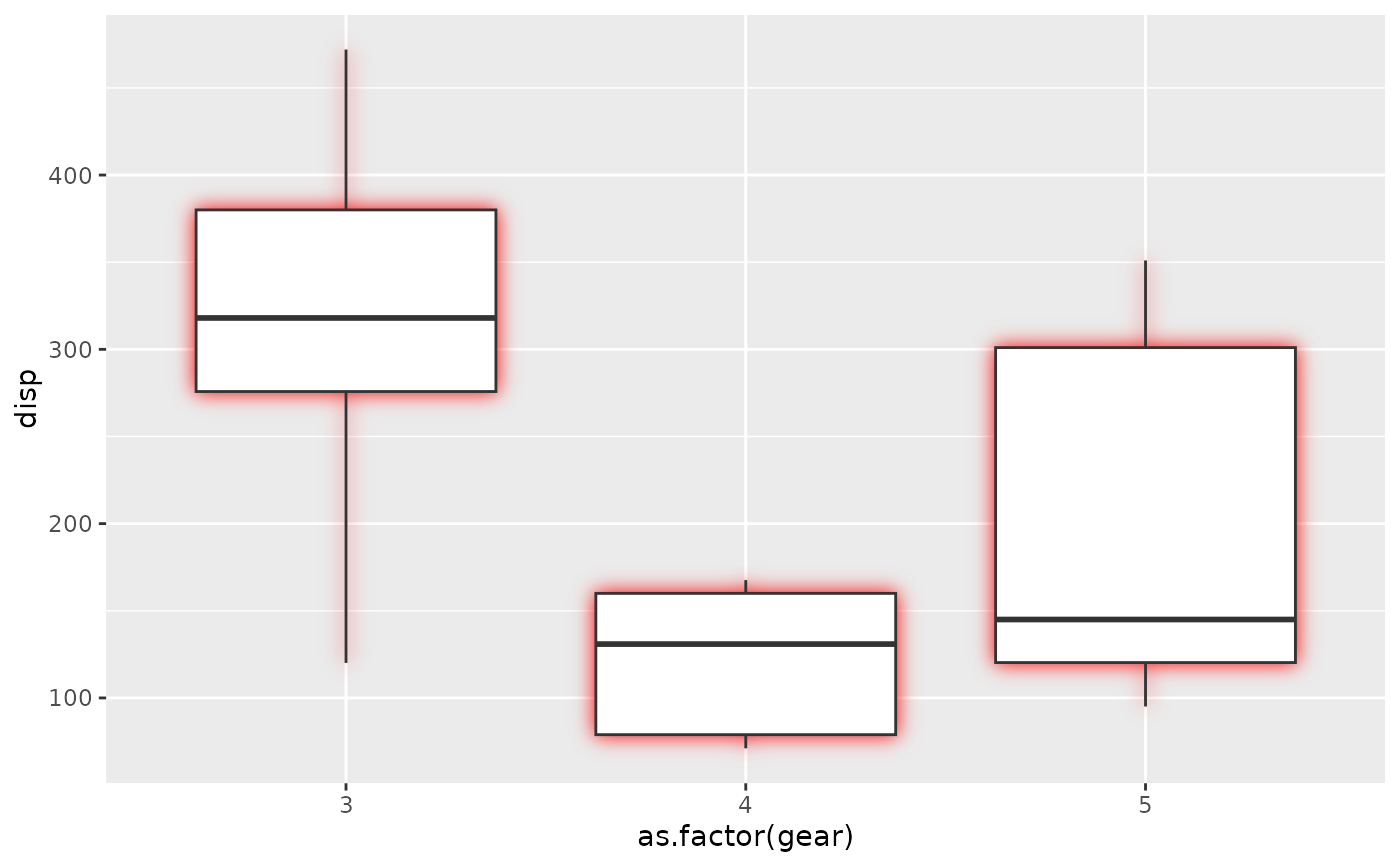This filter adds an outer glow to your layer with a specific colour and size. For very thin objects such as text it may be beneficial to add some expansion. See the examples for this.

with_outer_glow(x, colour = "black", sigma = 3, expand = 0, ...)

## Arguments

x

A ggplot2 layer object, a ggplot, a grob, or a character string naming a filter

colour

The colour of the glow

sigma

The standard deviation of the gaussian kernel. Increase it to apply more blurring. If a numeric it will be interpreted as given in pixels. If a unit object it will automatically be converted to pixels at rendering time

expand

...

Arguments to be passed on to methods. See the documentation of supported object for a description of object specific arguments.

## Value

Depending on the input, either a grob, Layer, list of Layers, guide, or element object. Assume the output can be used in the same context as the input.

Other glow filters: with_inner_glow()

## Examples

library(ggplot2)

ggplot(mtcars, aes(as.factor(gear), disp)) +
with_outer_glow(
geom_boxplot(),
colour = 'red',
sigma = 10
)# For thin objects (as the whiskers above) you may need to add a bit of
# expansion to make the glow visible:

ggplot(mtcars, aes(mpg, disp)) +
geom_point() +
with_outer_glow(
geom_text(aes(label = rownames(mtcars))),
colour = 'white',
sigma = 10,
expand = 10
)